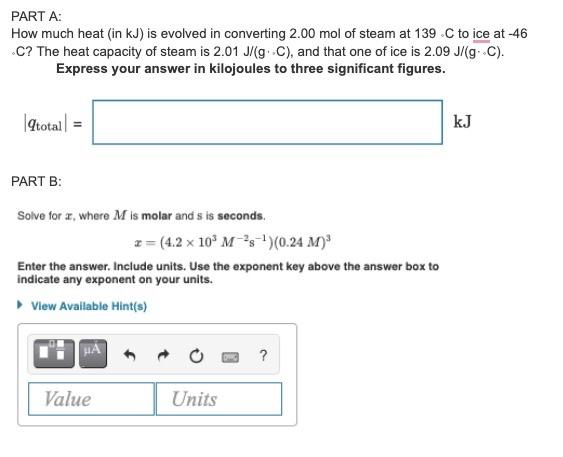# (Solved): need help with both parts and with steps please. thank you  need help please. this is 1 questio ...

need help with both parts and with steps please. thank youneed help please. this is 1 question since its the same chaper (11) and on the program it shows as part A-C but I only need help with part A and B.PART A: How much heat (in kJ) is evolved in converting $$2.00$$ mol of steam at $$139 . \mathrm{C}$$ to ice at $$-46$$ . C? The heat capacity of steam is $$2.01 \mathrm{~J} /(\mathrm{g} \cdot \mathrm{C})$$, and that one of ice is $$2.09 \mathrm{~J} /(\mathrm{g} \cdot \mathrm{C})$$. Express your answer in kilojoules to three significant figures. $\left|q_{\text {total }}\right|=$ PART B: Solve for $$x$$, where $$M$$ is molar and $$s$$ is seconds. $x=\left(4.2 \times 10^{3} M^{-2} \mathrm{~s}^{-1}\right)(0.24 M)^{3}$ Enter the answer. Include units. Use the exponent key above the answer box to indicate any exponent on your units. PART A: How much heat (in kJ) is evolved in converting $$2.00$$ mol of steam at $$139 . \mathrm{C}$$ to ice at $$-46$$ . C? The heat capacity of steam is $$2.01 \mathrm{~J} /(\mathrm{g} \cdot \mathrm{C})$$, and that one of ice is $$2.09 \mathrm{~J} /(\mathrm{g} \cdot \mathrm{C})$$. Express your answer in kilojoules to three significant figures. $\left|q_{\text {total }}\right|=$ PART B: Solve for $$x$$, where $$M$$ is molar and $$s$$ is seconds. $x=\left(4.2 \times 10^{3} M^{-2} \mathrm{~s}^{-1}\right)(0.24 M)^{3}$ Enter the answer. Include units. Use the exponent key above the answer box to indicate any exponent on your units.

We have an Answer from Expert